# Smart SONAR System

A Smart Sonar System which alerts the user when it spots objects or enemies within range.

IntermediateFull instructions provided12 hours499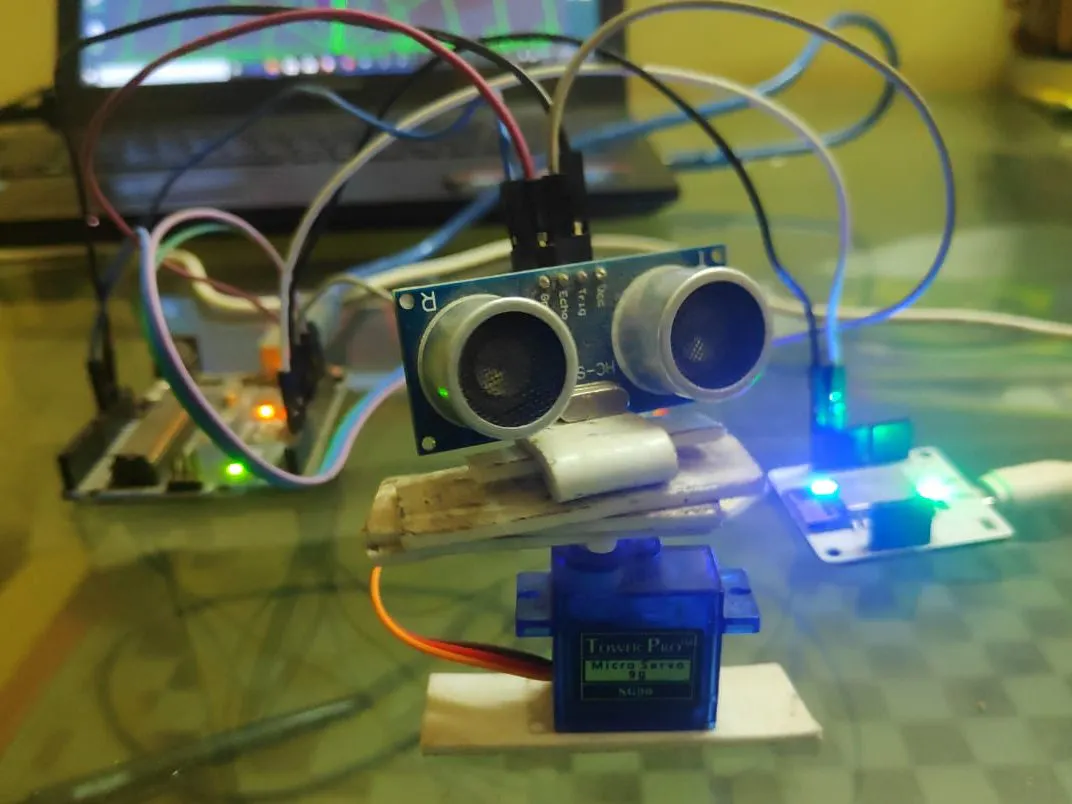## Things used in this project

### Hardware componentsArduino UNO & Genuino UNO
×1Ultrasonic Sensor - HC-SR04 (Generic)
×1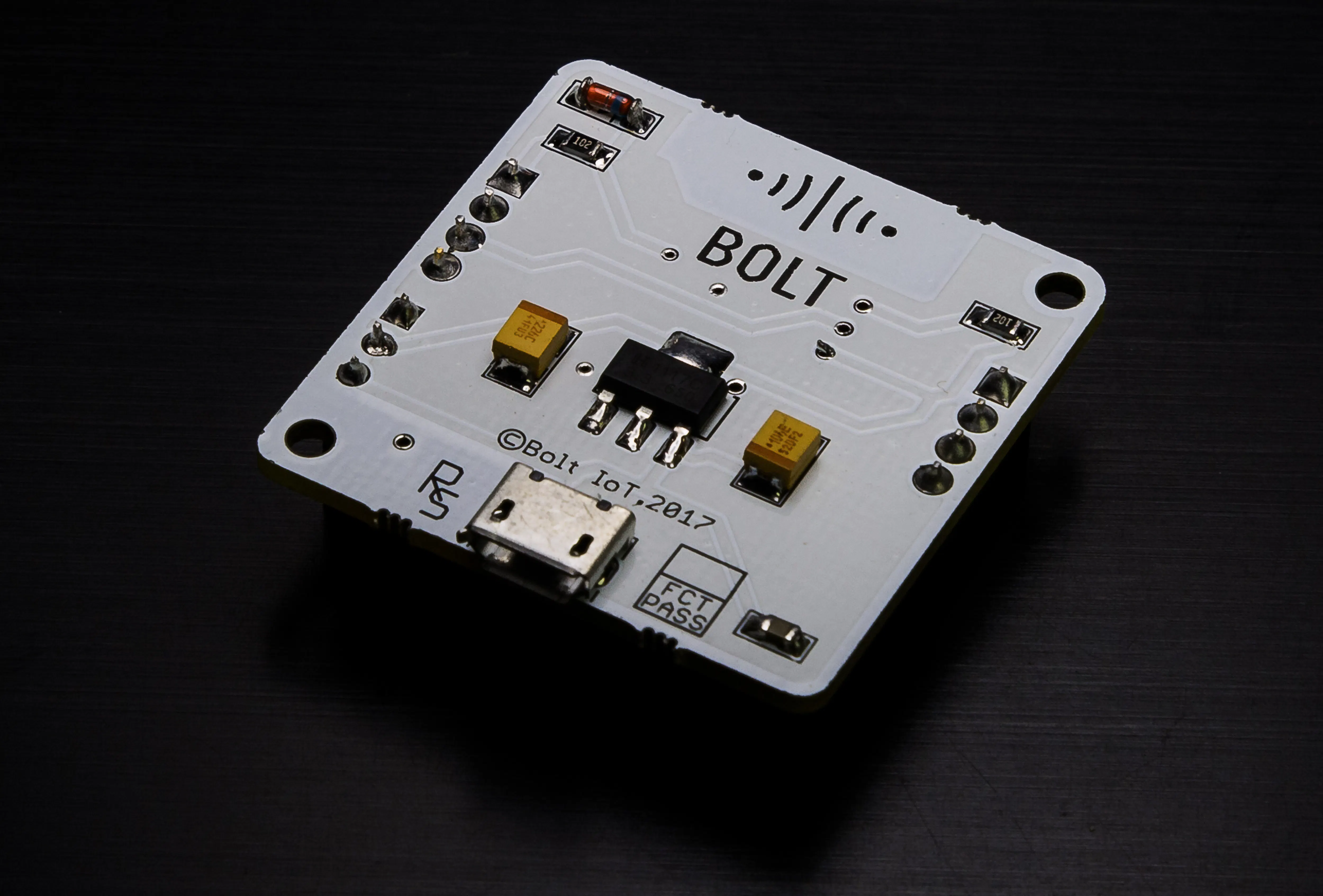Bolt IoT Bolt WiFi Module
×1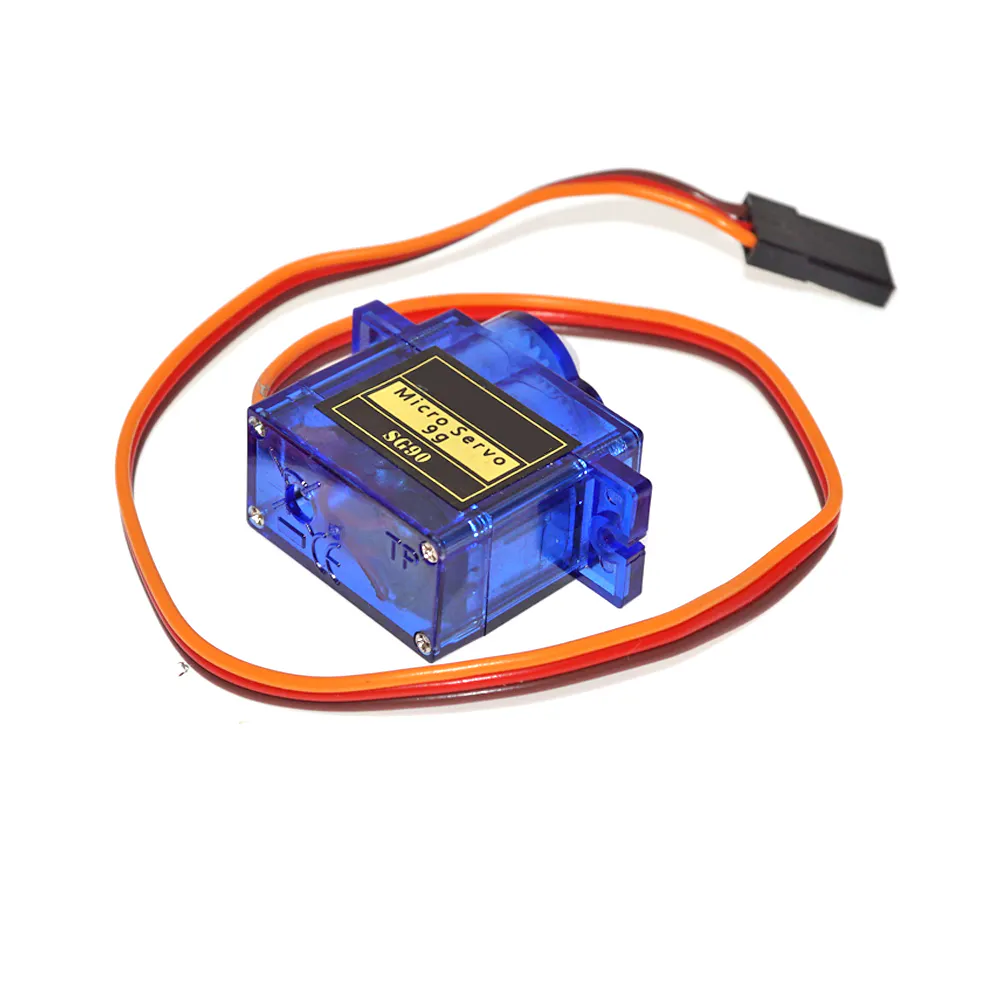SG90 Micro-servo motor
×1Jumper wires (generic)
×1×1

### Software apps and online servicesArduino IDEBolt IoT Bolt Cloud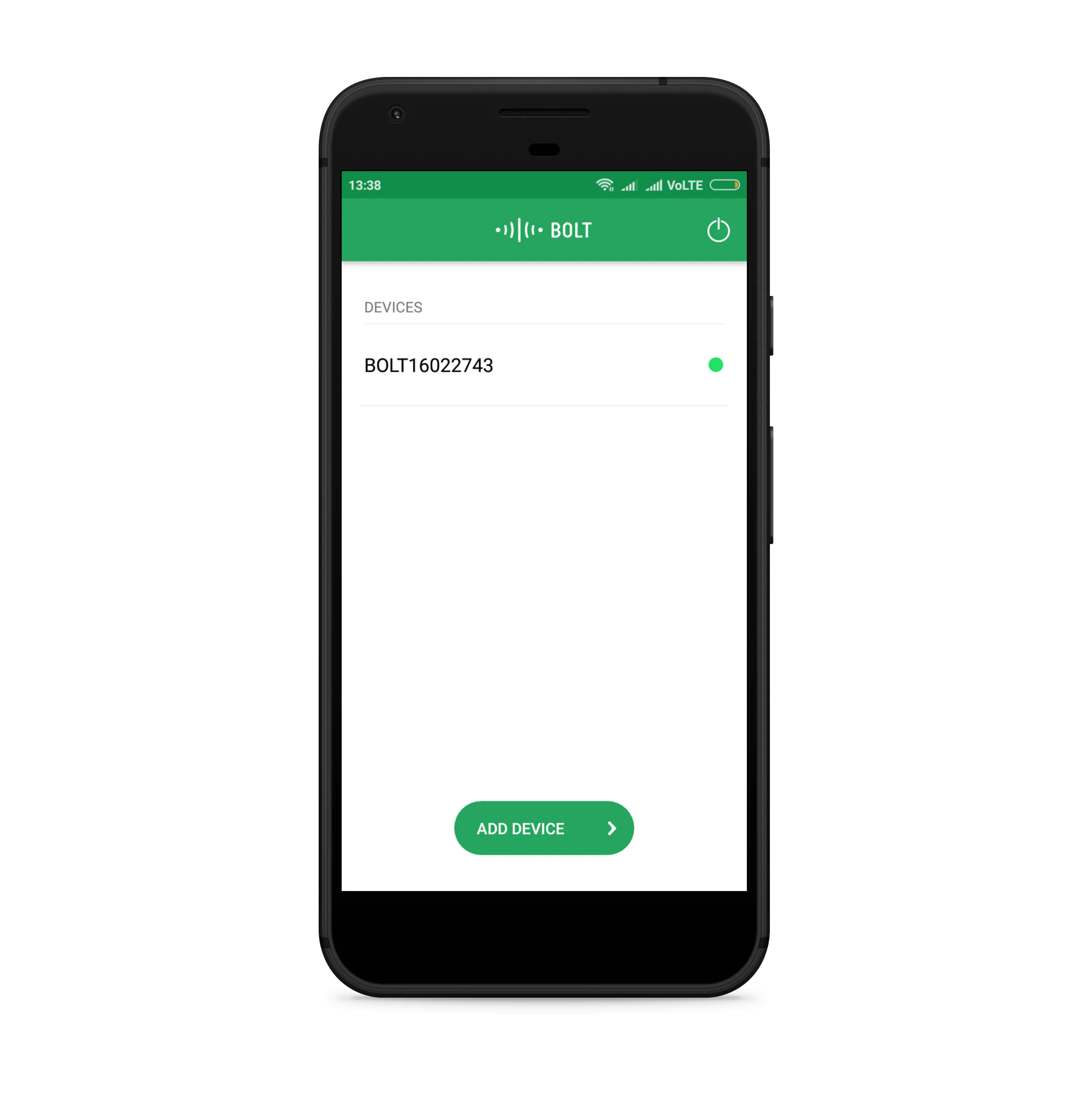Bolt IoT Android App
 Telegram MessagingThe Processing Foundation Processing

## Schematics

### Schematic SSS

A Schematic of the project on fritzing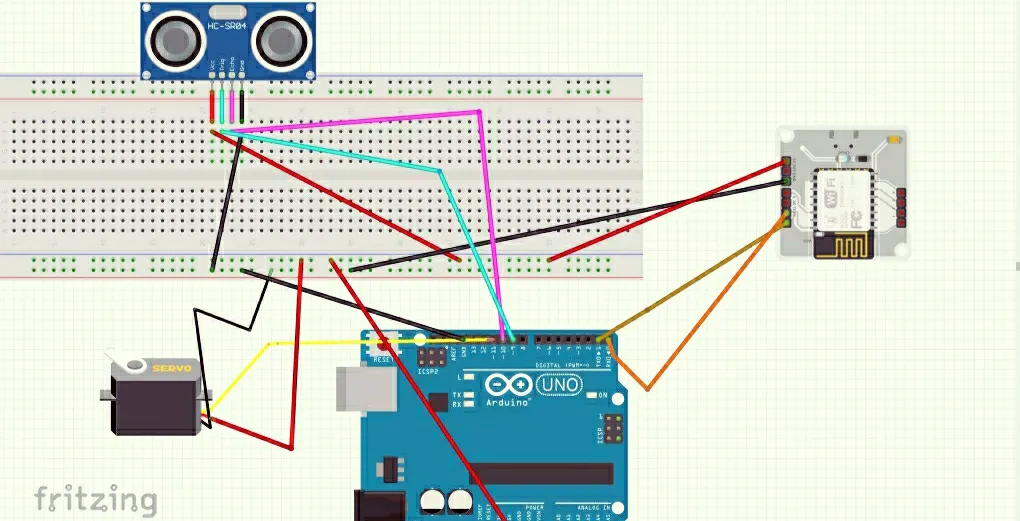## Code

### Telegram and Mailgun Code for Bolt IoT WiFi module

Python
Copy Paste in any OS having python inbuilt such as Ubuntu (terminal) or Linux OS (command line)
```from boltiot import Bolt,Email
import json, time, requests
import email_conf, conf
maximum_limit = 120 #the maximum threshold of distance value

mybolt = Bolt(email_conf.API_KEY, email_conf.DEVICE_ID)
mailer = Email(email_conf.MAILGUN_API_KEY, email_conf.SANDBOX_URL, email_conf.SENDER_EMAIL, email_conf.RECIPIENT_EMAIL)

def get_sensor_value_from_pin(pin):
"""Returns the sensor value. Returns -999 if request fails"""
try:
if data["success"] != 1:
print("Request not successfull")
print("This is the response->", data)
return -999
sensor_value = int(data["value"])
return sensor_value
except Exception as e:
print("Something went wrong when returning the sensor value")
print(e)
return -999
def send_telegram_message(message):
"""Sends message via Telegram"""
url = "https://api.telegram.org/" + conf.telegram_bot_id + "/sendMessage"
data = {
"chat_id": conf.telegram_chat_id,
"text": message
}
try:
response = requests.request(
"POST",
url,
params=data
)
print("This is the Telegram URL")
print(url)
print("This is the Telegram response")
print(response.text)
return telegram_data["ok"]
except Exception as e:
print("An error occurred in sending the alert message via Telegram")
print(e)
return False
while True:
print ("Sensor value is: " + str(data['value']))
try:
sensor_value = int(data['value'])
if sensor_value > maximum_limit:
print ("Making request to Mailgun to send an email")
response = mailer.send_email("Alert", "Object/Enemy is closing in! " +  str(conf.threshold) + \
". The current sensor  value is " +str(sensor_value))
print ("Response received from Mailgun is: " + str(response_text['message']))
if sensor_value >= conf.threshold:
print ("Sensor value has exceeded threshold")
message = "Alert! Sensor value has exceeded " + str(conf.threshold) + \
". The current value is " + str(sensor_value)
telegram_status = send_telegram_message(message)
print ("This is the Telegram status:", telegram_status)

if sensor_value == -999:
print ("Request was unsuccessfull. Skipping.")
time.sleep(10)
continue
except Exception as e:
print ("Error occured: Below are the details")
print (e)
time.sleep(10)
```

### Configuration code/file for Telegram

Python
```"""Configurations for Smart_Sonar.py"""
API_KEY = "XXXX"                 # This is your Bolt Cloud API Key
DEVICE_ID = "XXXX"                    # This is the device ID and will be similar to BOLTXXXX where XXXX is some numbers
telegram_chat_id = "@XXXX"            # This is the channel ID of the created Telegram channel. Paste after @ symbol.
telegram_bot_id = "botXXXX"           # This is the bot ID of the created Telegram Bot. Paste after bot text.
threshold = 120                      # Threshold beyond which the alert should be sent
```

### Arduino Code

Arduino
To control the servo motor and establish a connection between Bolt IoT WiFi module and Arduino and the sensor.
```// Includes the Servo library
#include <Servo.h>
#include <SoftwareSerial.h>
SoftwareSerial mySerial(0,1);
// Defines Tirg and Echo pins of the Ultrasonic Sensor
const int trigPin = 9;
const int echoPin = 10;
// Variables for the duration and the distance
long duration;
int distance;
Servo myServo; // Creates a servo object for controlling the servo motor
void setup() {
pinMode(trigPin, OUTPUT); // Sets the trigPin as an Output
pinMode(echoPin, INPUT); // Sets the echoPin as an Input
Serial.begin(9600);
myServo.attach(11); // Defines on which pin is the servo motor attached
}
void loop() {
// rotates the servo motor from 15 to 165 degrees
for(int i=15;i<=165;i++){
myServo.write(i);
delay(30);
distance = calculateDistance();// Calls a function for calculating the distance measured by the Ultrasonic sensor for each degree

Serial.print(i); // Sends the current degree into the Serial Port
Serial.print(","); // Sends addition character right next to the previous value needed later in the Processing IDE for indexing
Serial.print(distance); // Sends the distance value into the Serial Port
Serial.print("."); // Sends addition character right next to the previous value needed later in the Processing IDE for indexing
}
// Repeats the previous lines from 165 to 15 degrees
for(int i=165;i>15;i--){
myServo.write(i);
delay(30);
distance = calculateDistance();
Serial.print(i);
Serial.print(",");
Serial.print(distance);
Serial.print(".");
}
}
// Function for calculating the distance measured by the Ultrasonic sensor
int calculateDistance(){

digitalWrite(trigPin, LOW);
delayMicroseconds(2);
// Sets the trigPin on HIGH state for 10 micro seconds
digitalWrite(trigPin, HIGH);
delayMicroseconds(10);
digitalWrite(trigPin, LOW);
duration = pulseIn(echoPin, HIGH); // Reads the echoPin, returns the sound wave travel time in microseconds
distance= duration*0.034/2;
return distance;
}
```

### Processing App Code

Java
To visualize and graphically show the output.
```import processing.serial.*; // imports library for serial communication
import java.awt.event.KeyEvent; // imports library for reading the data from the serial port
import java.io.IOException;
Serial myPort; // defines Object Serial
// defubes variables
String angle="";
String distance="";
String data="";
String noObject;
float pixsDistance;
int iAngle, iDistance;
int index1=0;
int index2=0;
PFont orcFont;
void setup() {

size (1200, 700); // ***CHANGE THIS TO YOUR SCREEN RESOLUTION***
smooth();
myPort = new Serial(this,"COM3", 9600); // starts the serial communication
myPort.bufferUntil('.'); // reads the data from the serial port up to the character '.'. So actually it reads this: angle,distance.
}
void draw() {

fill(98,245,31);
// simulating motion blur and slow fade of the moving line
noStroke();
fill(0,4);
rect(0, 0, width, height-height*0.065);

fill(98,245,31); // green color
// calls the functions for drawing the radar
drawLine();
drawObject();
drawText();
}
void serialEvent (Serial myPort) { // starts reading data from the Serial Port
// reads the data from the Serial Port up to the character '.' and puts it into the String variable "data".
data = data.substring(0,data.length()-1);

index1 = data.indexOf(","); // find the character ',' and puts it into the variable "index1"
angle= data.substring(0, index1); // read the data from position "0" to position of the variable index1 or thats the value of the angle the Arduino Board sent into the Serial Port
distance= data.substring(index1+1, data.length()); // read the data from position "index1" to the end of the data pr thats the value of the distance

// converts the String variables into Integer
iAngle = int(angle);
iDistance = int(distance);
}
pushMatrix();
translate(width/2,height-height*0.074); // moves the starting coordinats to new location
noFill();
strokeWeight(2);
stroke(98,245,31);
// draws the arc lines
arc(0,0,(width-width*0.0625),(width-width*0.0625),PI,TWO_PI);
arc(0,0,(width-width*0.27),(width-width*0.27),PI,TWO_PI);
arc(0,0,(width-width*0.479),(width-width*0.479),PI,TWO_PI);
arc(0,0,(width-width*0.687),(width-width*0.687),PI,TWO_PI);
// draws the angle lines
line(-width/2,0,width/2,0);
popMatrix();
}
void drawObject() {
pushMatrix();
translate(width/2,height-height*0.074); // moves the starting coordinats to new location
strokeWeight(9);
stroke(255,10,10); // red color
pixsDistance = iDistance*((height-height*0.1666)*0.025); // covers the distance from the sensor from cm to pixels
// limiting the range to 40 cms
if(iDistance<40){
// draws the object according to the angle and the distance
}
popMatrix();
}
void drawLine() {
pushMatrix();
strokeWeight(9);
stroke(30,250,60);
translate(width/2,height-height*0.074); // moves the starting coordinats to new location
popMatrix();
}
void drawText() { // draws the texts on the screen

pushMatrix();
if(iDistance>40) {
noObject = "Out of Range";
}
else {
noObject = "In Range";
}
fill(0,0,0);
noStroke();
rect(0, height-height*0.0648, width, height);
fill(98,245,31);
textSize(25);

text("10cm",width-width*0.3854,height-height*0.0833);
text("20cm",width-width*0.281,height-height*0.0833);
text("30cm",width-width*0.177,height-height*0.0833);
text("40cm",width-width*0.0729,height-height*0.0833);
textSize(40);
text("Indian Lifehacker ", width-width*0.875, height-height*0.0277);
text("Angle: " + iAngle +" °", width-width*0.48, height-height*0.0277);
text("Distance: ", width-width*0.26, height-height*0.0277);
if(iDistance<40) {
text("        " + iDistance +" cm", width-width*0.225, height-height*0.0277);
}
textSize(25);
fill(98,245,60);
text("30°",0,0);
resetMatrix();
text("60°",0,0);
resetMatrix();
text("90°",0,0);
resetMatrix();
text("120°",0,0);
resetMatrix();
text("150°",0,0);
popMatrix();
}
```

### Configuration code/file for Mailgun

Python
```MAILGUN_API_KEY = 'This is the private API key which you can find on your Mailgun Dashboard'
SANDBOX_URL= 'You can find this on your Mailgun Dashboard'
SENDER_EMAIL = 'This would be test@your SANDBOX_URL'
RECIPIENT_EMAIL = 'Enter your Email ID Here'
API_KEY = 'This is your Bolt Cloud account API key'
DEVICE_ID = 'This is the ID of your Bolt device'
```

## Credits

### Pranay Pandit

0 projects • 0 followers